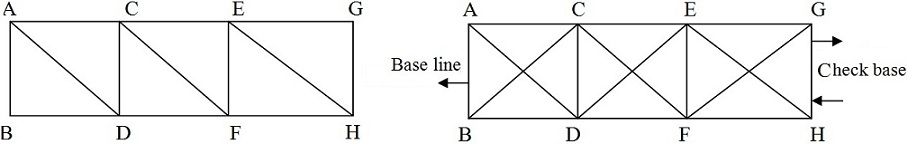Feb 26, 2016

Feb 26, 2016

Feb 26, 2016

# How to do Trigonometric Surveying

## Definition:

Geodetic or trigonometrically surveying takes into account the curvature of earth Since very extensive areas and very large distances are involved. In geodetic surveying highly refined instruments and methods are used. Geodetic work is undertaken by the state agency e.g. survey of Pakistan undertaken by the state agency.

1. Triangulation
2. Precise leveling

## Object:

The object of geodetic surveying is to accurately determine the relative position of a sys of widely separated pts (stations) on the surface of earth and also their absolute positions.

Relative positions are determined in terms of azimuths and length f lines joining them. Absolute positions are determined in terms of latitude and longitudes and elevations above mean sea laves. The methods employed in geodetic surveying are:

1. Triangulation (most accurate but expensive)
2. Precise traverse (inferior and used when triangulation is physically impossible or very expensive) e.g. Densely wooded country.

## Triangulation:

It is based on the trigonometry proposition that of one side and three angles be computed by the application of since rule. In this method suitable points called triangulation stations are selected and established throughout the area to be surveyed.

The stations may be connected by a series of triangles or a chain of quadrilaterals as shown.### Baseline:

Whose length is measured these stations form the vertices of a series mutually connected, triangles the complete figure being called ‘Triangulation system’. In this system of triangles one line say ‘AB’ and all the angles are measured with greatest care and lengths of all the remaining line in the system are then computed. For checking both the fieldwork and computation another line say GH is very accurately measured at the end of the system. The line whose length is actually measured is known as baseline or base and that measured for checking purpose is known as the check base.

### Triangulation Figures:

The geometric figures used in triangulation system are (i) Triangles (ii) Quadrilaterals (ii) Quadrilaterals, Pentagon, hexagons with centre angle. This arrangement although simple and economical but less accurate since the number of conditions involve in its adjustment is small.

1. Station adjustment ==> sum of angle is 180
2. Figure adjustment ==> sum of angles is 400 grad or 360Quadrilaterals pentagons or hexagonal with central stations. For very accurate work a chain of quadrilaterals may be used. There is no station at the intersection of diagonals. This system is most accurate since the number of conditions in its adjustments is much greater. To minimize the effect of small errors in measurement of angles the triangles hold be well shaped or well proportioned i.e. they should not have angle less than 30 or greater than 120.The best shape triangle is equilaterals triangle and best shape quadrilateral is square.